Untitled Document Untitled Document
Untitled Document Untitled Document
Untitled Document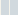1.  The goal is to analyze a KYB fork.  First thing is to search for the test.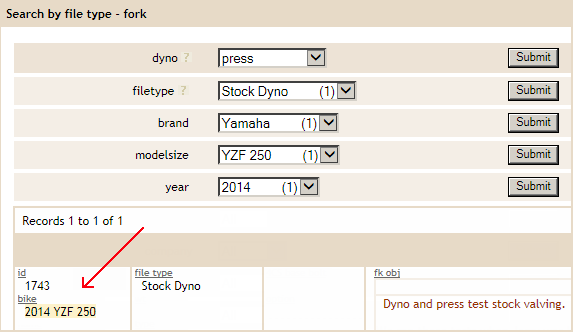2.  Next we go to the Fork Valving page and view the spec sheet.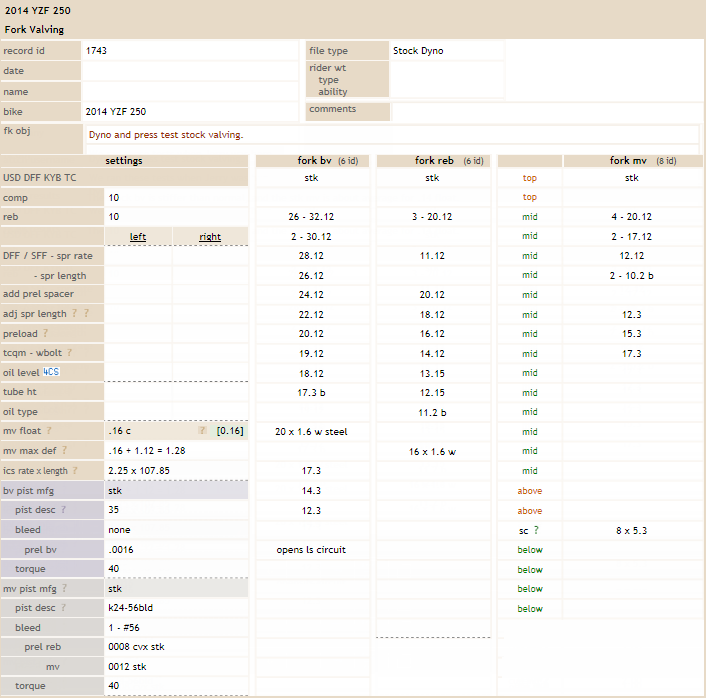3.  Then click the Fork Dyno tab to view dyno data.     [How to Analyze Dyno Data]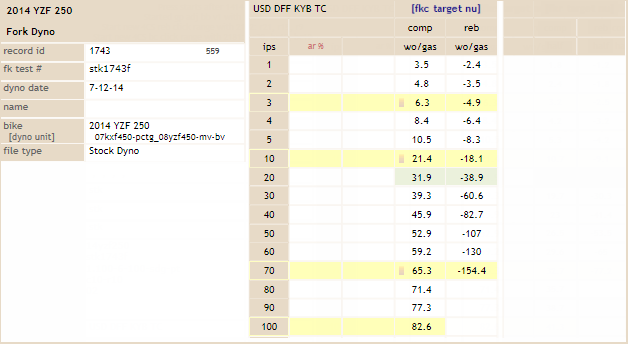4.  Click the Fork Pressure tab to view the breakdown of compression forces.
For example, we can determine:
-- how much is bv force
-- how much is mv force
-- how much is gas force  (from inner chamber spring  [icspr])
-- how much is drag force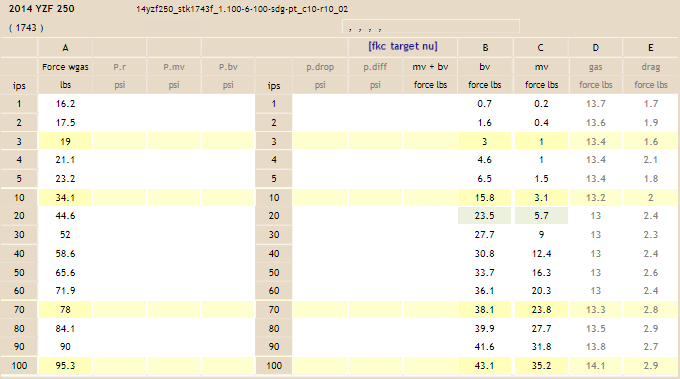5. This is where it gets interesting.  Column B (from above) shows the bv force and column C shows the mv force.  This shows the relationship between the bv and mv, and can see how much work each is doing at the different velocities.  In other words, for any given velocity, we want to consider how much force is coming from the bv and how much is coming from the mv.  Knowing these forces are the key to balancing/optimizing the bv and mv stacks. Once you pressure test and compare enough bikes, you begin to see a pattern.  For example, one thing we noticed for amateur MX is that all forks produce about the same compression and rebound damping forces.  Whether it's a 125, 250 or 450, the dyno numbers are all very close and fit within a tight range.  At the beginning this allowed us to define a scale for the fork compression forces calling them soft-average-stiff, with 15% change between the ranges.  It didn't take long and we expanded the range from soft-6 for Enduro to stiff+9 for SX.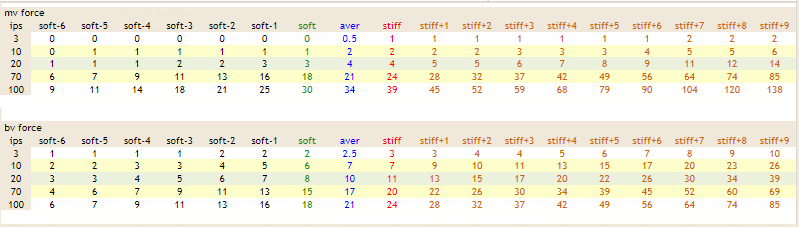---> This is the  'fork compression target number' scale  [fkc target nu].

 6. Using the scale above, we can rank the 2014 YZF 250 mv and bv numbers.  Rounding and comparing the numbers at 70ips.    -- mv force = 23.8 lbs = approx stiff    -- bv force = 38.1 lbs = approx stiff+5 This shows exactly how this fork compares with other forks and how the bv force compares with the mv force.  Proportionately, the yzf250 bv force is higher than the mv force .  We're not saying this is good or bad, we just have a way to see how they relate. The scale is comparable for forks from different manufacturers.  It doesn't make any difference if they have different piston diameters, rod diameters etc that are found in forks.  Pressure testing shows the final output of the combined components in the fork.  Once assembled and tested, all fork compression numbers are going to fit the scale. You can see how this simplifies the tuning process.  You simply pressure test any fork setting, see where the bv and mv forces fit in the scale, and adjust accordingly.  Through extensive testing we have found what we consider to be ideal 'target numbers' for the bv and mv force, depending on the application.  When a new bike comes in, we valve the fork accord to our current recommended 'target numbers' and let the rider test.  If changes are needed, it is easy to adjust the bv or mv stack as needed, depending on rider feedback. This is an ongoing process.  Each time we make an adjustment, our target number scale improves.  Because we have a definitive way to compare the valving configurations, the process steadily becomess easier and more precise. We will conclude with an example of one extreme difference between bv and mv force.  We received a SX fork that lost all its compression damping.  Upon inspection, we noticed the bv icrod was bent.  After replacing the rod and pressure testing the configuration we saw the bv icrod bent because the bv force was very high (apparently putting side loads on the piston bending the rod).     -- mv force = stiff+2     -- bv force = stiff+14  (off the scale)     --> This was very unbalanced. We revalved and opted for:     -- mv force = stiff+7     -- bv force = stiff+8  The rider rode the forks and said they worked very well.

7.  We have a similar method for analyzing and comparing shocks.

THE END